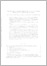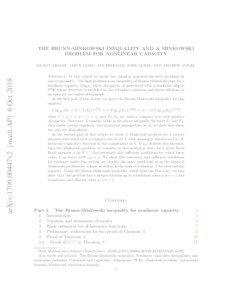# The Brunn-Minkowski inequality and A Minkowski problem for nonlinear capacity

Akman, Murat and Gong, Jasun and Hineman, Jay and Lewis, John and Vogel, Andrew (2018) 'The Brunn-Minkowski inequality and A Minkowski problem for nonlinear capacity.' Memoirs of the American Mathematical Society. ISSN 0065-9266 (In Press)Preview
Text
1709.00447v2.pdf - Submitted Version

In this article we study two classical potential-theoretic problems in convex geometry. The first problem is an inequality of Brunn-Minkowski type for a nonlinear capacity, $\mbox{Cap}_{\mathcal{A}}$, where $\mathcal{A}$-capacity is associated with a nonlinear elliptic PDE whose structure is modeled on the $p$-Laplace equation and whose solutions in an open set are called $\mathcal{A}$-harmonic. In the first part of this article, we prove the Brunn-Minkowski inequality for this capacity: $\left[\mbox{Cap}_\mathcal{A} ( \lambda E_1 + (1-\lambda) E_2 )\right]^{\frac{1}{(n-p)}} \geq \lambda \, \left[\mbox{Cap}_\mathcal{A} ( E_1 )\right]^{\frac{1}{(n-p)}} + (1-\lambda) \left[\mbox{Cap}_\mathcal{A} (E_2 )\right]^{\frac{1}{(n-p)}}$ when $1 < p < n$, $0 < \lambda < 1$, and $E_1, E_2$ are convex compact sets with positive $\mathcal{A}$-capacity. Moreover, if equality holds in the above inequality for some $E_1$ and $E_2,$ then under certain regularity and structural assumptions on $\mathcal{A},$ we show that these two sets are homothetic. In the second part of this article we study a Minkowski problem for a certain measure associated with a compact convex set $E$ with nonempty interior and its $\mathcal{A}$-harmonic capacitary function in the complement of $E$. If $\mu_E$ denotes this measure, then the Minkowski problem we consider in this setting is that; for a given finite Borel measure $\mu$ on $\mathbb{S}^{n-1}$, find necessary and sufficient conditions for which there exists $E$ as above with $\mu_E = \mu$. We show that necessary and sufficient conditions for existence under this setting are exactly the same conditions as in the classical Minkowski problem for volume as well as in the work of Jerison for electrostatic capacity. Using the Brunn-Minkowski inequality result from the first part, we also show that this problem has a unique solution up to translation when $p\neq n- 1$ and translation and dilation when $p = n-1$.View Item